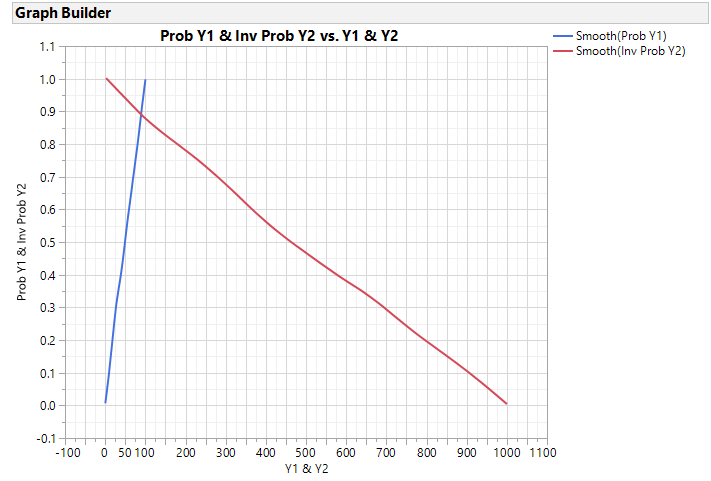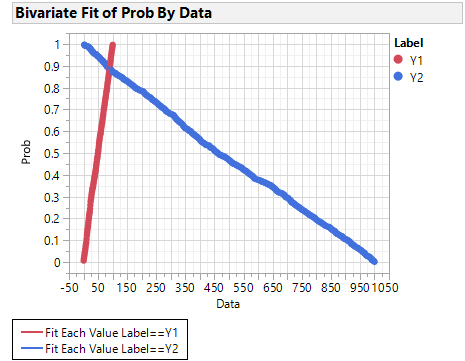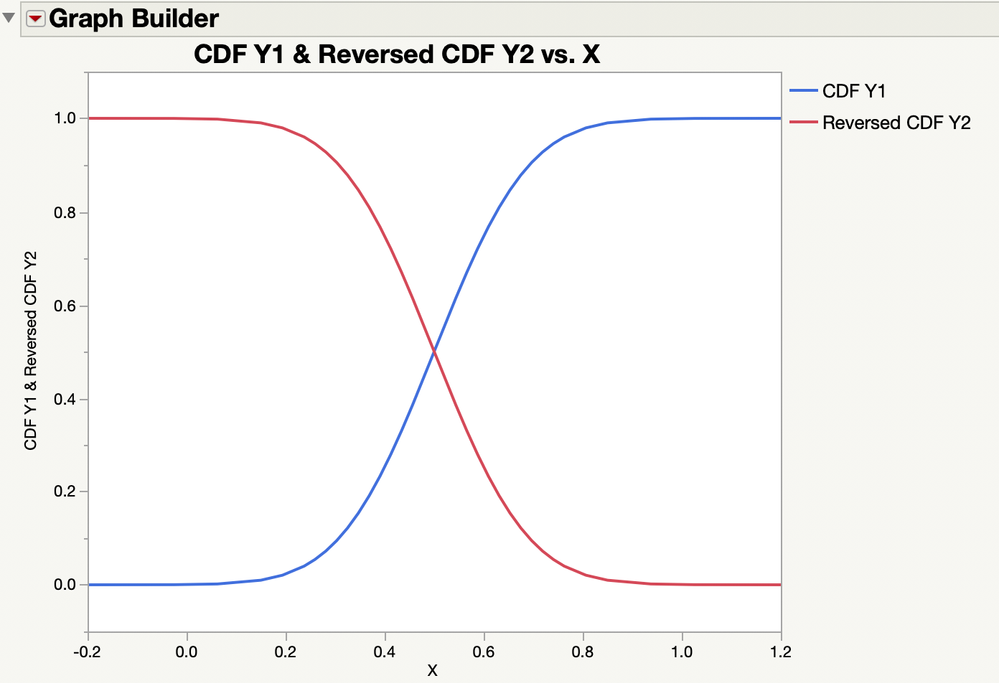World Statistics Day was yesterday, but we’re celebrating all week long! This celebration means acknowledging the impact statistics has on our world. Who is your favorite statistician? Share with us who they are and why they top your favorites list.
Choose Language Hide Translation Bar
Highlighted

## Plot conventional and reverse CDF on same plot by grouping variable

Hello,

Have a question on CDF plots on the distribution platform

I would like to plot the CDF of two variables say Y1 and Y2 which are on the same numerical scale but differ by the range/. For example Y1 from 0-100 and Y2 from 0 to 1000. I want to plot Y1like a regular CDF plot with the CumProb going from 0 to 1 and X1 values increasing from 0 to 100.

For Y2, I want the X2 to be in decreasing order from 1000 to 0 but the cumprob of Y2 runs from 0 to Y increasing in the Y axis direction.

I want to essentially reverse cdf(Y2) and overplot with cdf(Y1) and plot both on the same cdf plot window. Eventually I want to shade specific areas under the curves based on X1 and X2 values but before that I want to know how I could accomplish this task?

I started with getting the probability score and adding a column for 1-P(X2 > x) for Y2 but was little stuck on how I could get 1-P(X2>x) and P(X1<=x1) on the same X axis and was running around circles with how I could approach this in general. Any thoughts and pointers would be really helpful. I have 5 different categories of a grouping variable and do not mind having 5 different such cdf plots.

2 REPLIES 2
Highlighted

## Re: Plot conventional and reverse CDF on same plot by grouping variable

Attached is a script that I believe simulates your data setup and two methods to create the graph you described. One uses unstacked data ( two columns) and another with stacked data.  If you are looking for rows where the Inv Prob of Y2 > Prob Y1 the unstacked (raw) data would be easier to use.

Note, Y1 and Y2 were simulated as uniform distributions.

``````Names Default to Here(1);

dt = New Table("Demo - Raw", Add rows(1000),
New Column("Y1", Numeric, Continuous, <<Set Each Value(Random Integer(0,100)) ),
New Column("Y2", Numeric, Continuous, <<Set Each Value(Random Integer(0,1000)) )
);

dist = dt << Distribution(
Continuous Distribution( Column( :Y1 ) ),
Continuous Distribution( Column( :Y2 ) )
);

dist << Save(Prob Scores);

dist << close window();

dt << New Column("Inv Prob Y2", numeric, continuous, <<Set Each Value(1-:Prob Y2));

//Using Unstacked data and GraphBuilder
gb = dt << Graph Builder(
Size( 534, 454 ),
Show Control Panel( 0 ),
Variables(
X( :Y1 ),
X( :Y2, Position( 1 ) ),
Y( :Prob Y1 ),
Y( :Inv Prob Y2, Position( 1 ) )
),
Elements(
Smoother( X( 1 ), Y( 1 ), Legend( 25 ) ),
Smoother( X( 2 ), Y( 2 ), Legend( 27 ) )
),
SendToReport(
Dispatch(
{},
"Y1",
ScaleBox,
{Min( -100 ), Max( 1100 ), Inc( 50 ), Minor Ticks( 1 ),
Label Row( {Show Major Grid( 1 ), Show Minor Grid( 1 )} )}
),
Dispatch(
{},
"Prob Y1",
ScaleBox,
{Min( -0.1 ), Max( 1.1 ), Inc( 0.1 ), Minor Ticks( 1 ),
Label Row( {Show Major Grid( 1 ), Show Minor Grid( 1 )} )}
)
)
);

//Sometimes it is easier to stack the data and use Bivariate instead of GraphBuilder

dtstck = dt << Stack(
columns( :Y1, :Y2, :Prob Y1, :Inv Prob Y2 ),
Source Label Column( "Label" ),
Stacked Data Column( "Data" ),
Stack By Row( 0 ),
Number of Series( 2 ),
Contiguous,
Output Table Name("Demo - Stacked")
);

dtstck:Data2 << set name("Prob");

//this creates one graph with 2 curves
biv = dtstck << Bivariate(
Y( :Prob ),
X( :Data ),
Group By(:Label),
Fit Each Value( {Report(0)}),
SendToReport(
Dispatch(
{},
"1",
ScaleBox,
{Min( -50 ), Max( 1050 ), Inc( 50 ), Minor Ticks( 1 ),
Label Row( {Show Major Grid( 1 ), Show Minor Grid( 1 )} )}
),
Dispatch(
{},
"2",
ScaleBox,
{Label Row( {Show Major Grid( 1 ), Show Minor Grid( 1 )} )}
),

Dispatch(
{},
"Bivar Plot",
FrameBox,
{Row Legend(
Label,
Color( 1 ),
Color Theme( "JMP Default" ),
Marker( 0 ),
Marker Theme( "" ),
Continuous Scale( 0 ),
Reverse Scale( 0 ),
Excluded Rows( 0 )
)}
)
)
);
``````

Here are the two graphs:Highlighted

## Re: Plot conventional and reverse CDF on same plot by grouping variable

I like Georgia's solution a lot. Here is a simpler and different approach: normalizing the two data sets before combining the plot. It might not be as satisfying.

I used two normal distributions with different parameters to illustrate this approach. You could save the fitted model for a distribution of sample data as a column formula instread of making up the data as I did. Here is the resulting plot:I attached the data table that I made to produce this plot.

Learn it once, use it forever!
Article Labels

There are no labels assigned to this post.